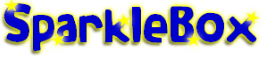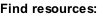﻿ KS2 Times Tables Teaching Resources and Printables - SparkleBox

HOME > Maths > Calculations > Times Tables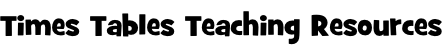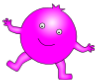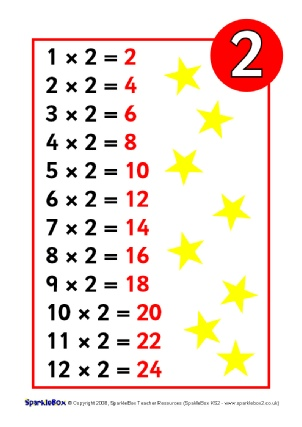Times Tables Posters (SB6597)

A set of printable posters showing the times tables from 2 up to 12.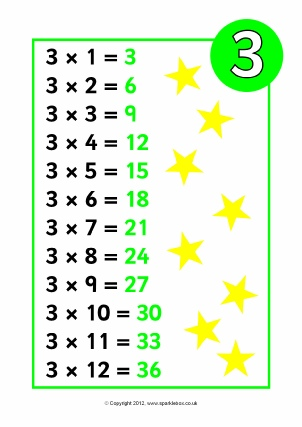Times Tables Posters - Reversed (SB7703)

A set of printable posters showing the times tables from 2 up to 12.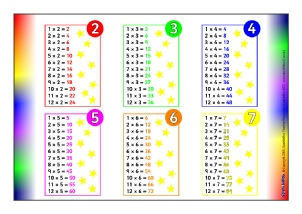Times Tables Mats (SB6598)

Two printable mats (which could be printed double-sided) showing the times tables.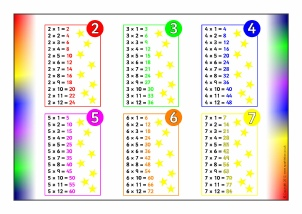Times Tables Mats - Reversed (SB7704)

Two printable mats (which could be printed double-sided) showing the times tables.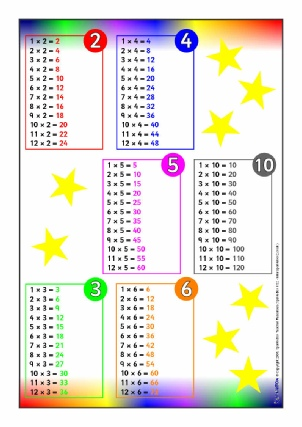Times Tables Mat 2 (SB6599)

A simple printable times table mat featuring 2 & 4, 3 & 6 and 5 & 10 times tables.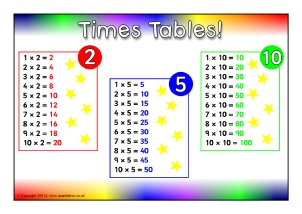2, 5 and 10 Times Tables Mat (SB9060)

A simple printable times table mat featuring 2 5 and 10 times tables.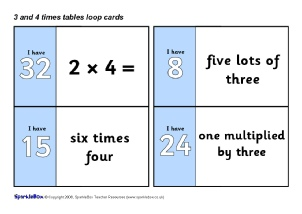3 and 4 Times Table Loop Cards (SB6604)

A set of 20 printable loop cards which can be used in small groups or with pairs.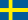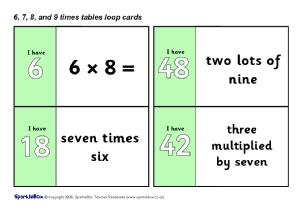6, 7, 8 and 9 Times Table Loop Cards (SB6605)

A set of loop cards with the 6, 7, 8 and 9 multiplication tables.  31 different cards included.  Great for whole-class or smaller-group phonics game where one child calls out the start card and the pupils then match/call out the corresponding cards until the game ‘loops’ back to the original child.

Know Your Times Tables Display Banners (SB8800)

Colour banners for your classroom times tables board.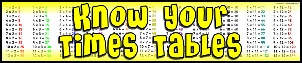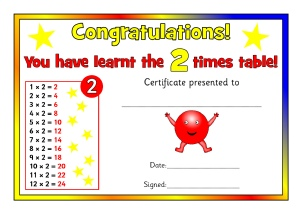Times Tables Certificates (SB8987)

A set of colourful certificates to present to your pupils when they have learnt each of the times tables.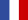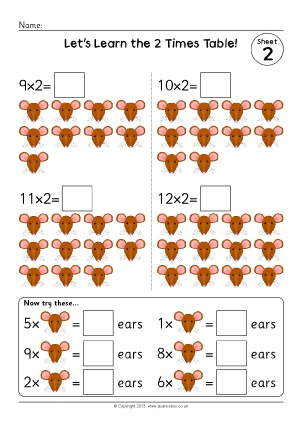2 Times Table Worksheets (SB9379)

A set of simple worksheets where children count the items and complete 2 times table number sentences.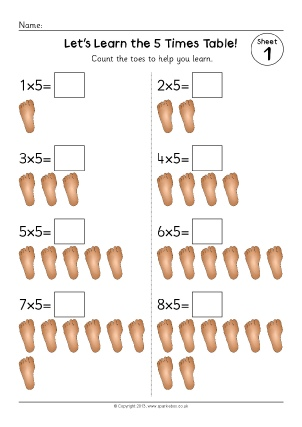5 Times Table Worksheets (SB9382)

A set of simple worksheets where children count the items and complete 3 times table number sentences.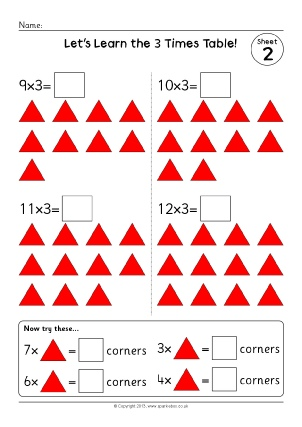3 Times Table Worksheets (SB9386)

A set of simple worksheets where children count the items and complete 3 times table number sentences.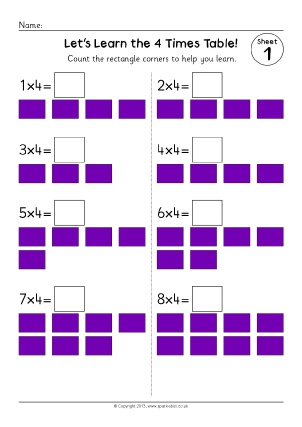4 Times Table Worksheets (SB9396)

A set of simple worksheets where children count the corners and complete 4 times table number sentences.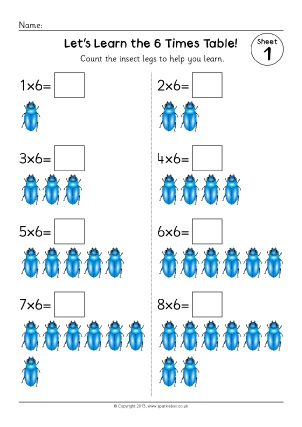6 Times Table Worksheets (SB9402)

A set of simple worksheets where children count the insect legs and complete 6 times table number sentences.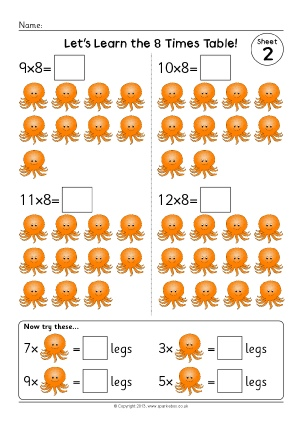8 Times Table Worksheets (SB9410)

A set of simple worksheets where children count the items and complete 8 times table number sentences.

Resizable Multiplication Square Image (SB6601)

A downloadable multiplication square JPG image which can used in your own worksheets, posters or PowerPoints etc.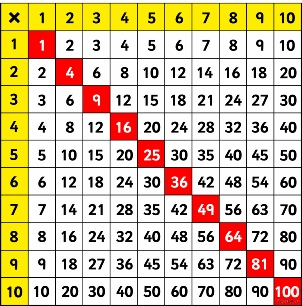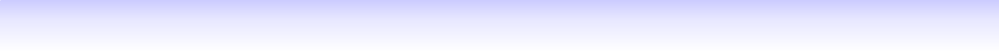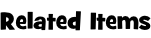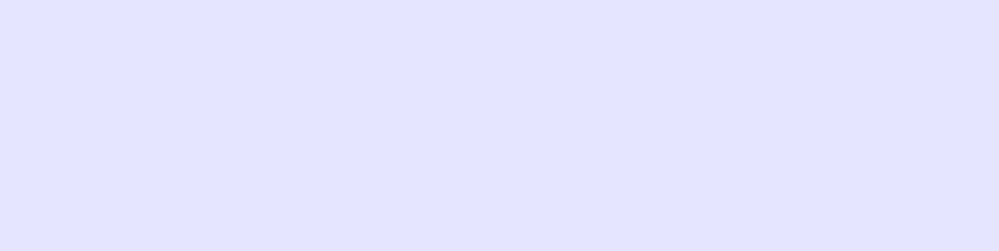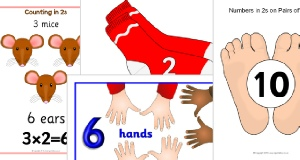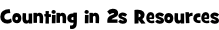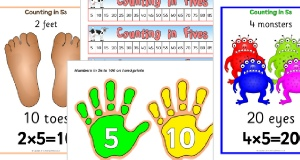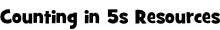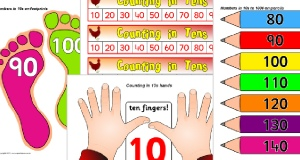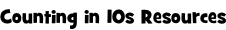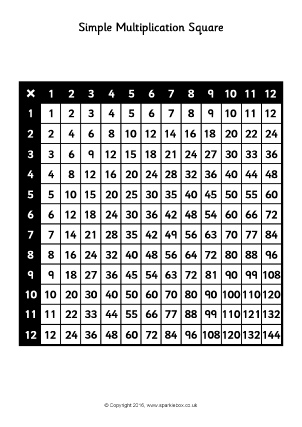Simple Multiplication Square Sheets (SB11741)

Simple printable multiplication squares in colour and black and white up to 10x and 12x tables.  Useful for all sorts of classroom activities.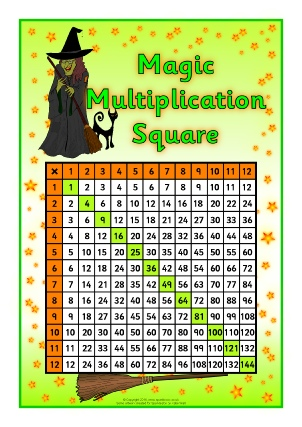Witch and Wizard-Themed Multiplication Square Sheets (SB11740)

Print out and laminate these colourful multiplication square sheets to use in all sorts of classroom activities.  Includes 10x and 12x tables versions.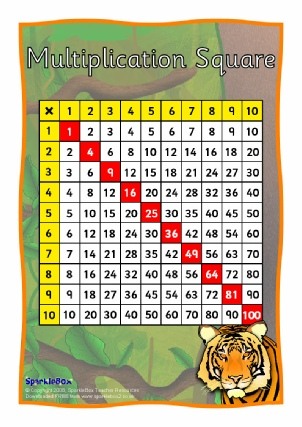Multiplication Square Sheets (SB6600)

Print out and laminate these colourful multiplication square sheets for use as table-top references.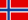Giant Multiplication Square Pictures for Display (SB11742)

Large printable multiplication squares that print over 12 portrait A4 pages.  In both colour and black and white, 10x and 12x tables.  Assemble these on your classroom display for great visual impact!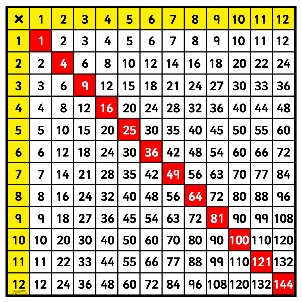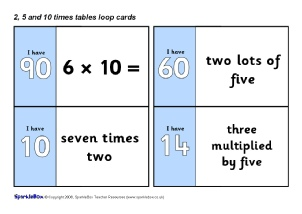2, 5 and 10 Times Table Loop Cards (SB1241)

A set of 24 printable loop cards which can be used in small groups or with pairs.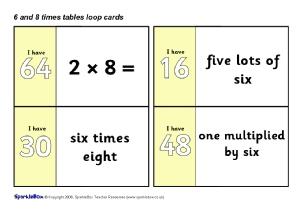6 and 8 Times Table Loop Cards (SB6603)

A set of 20 printable loop cards which can be used in small groups or with pairs.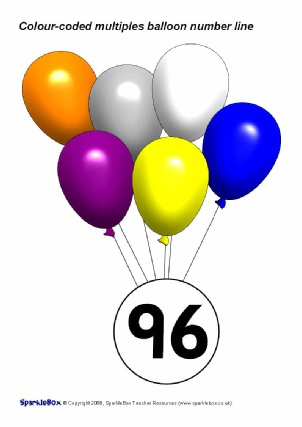Colour-Coded Multiples with Balloons (SB1911)

The numbers from 1-100 with colour-coded balloons to show multiples of 2, 3, 4, 5, 6, 7, 8, 9 and 10 and odd/even numbers.  A useful visual aid for your classroom.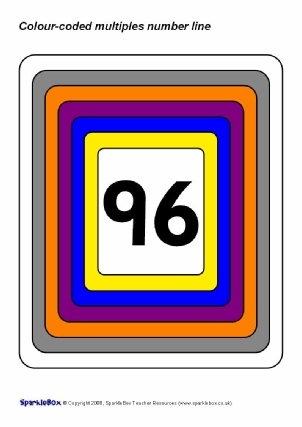Colour-Coded Multiples Number Line (SB1910)

The numbers from 1-100 colour-coded to show multiples of 2, 3, 4, 5, 6, 7, 8, 9 and 10 and odd/even numbers.  A useful visual aid for your classroom.

Counting in
10sCounting in
11sCounting in
12sCounting in
100s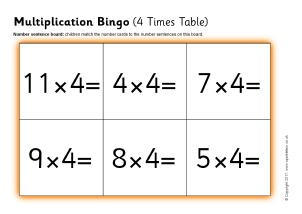Multiplication Bingo (4 Times Table) (SB12042)

A set of printable bingo boards and number cards with multiplication number sentences from the 4 times table.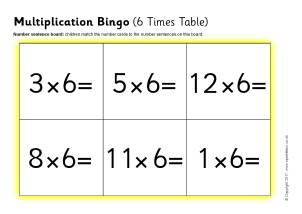Multiplication Bingo (6 Times Table) (SB12041)

A set of printable bingo boards and number cards with multiplication number sentences from the 6 times table.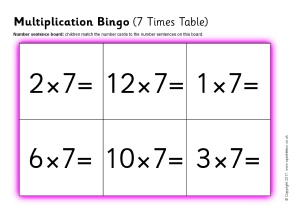Multiplication Bingo (7 Times Table) (SB12046)

A set of printable bingo boards and number cards with multiplication number sentences from the 7 times table.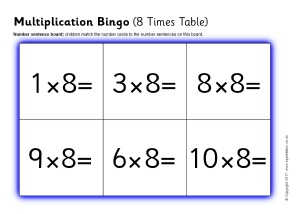Multiplication Bingo (8 Times Table) (SB12047)

A set of printable bingo boards and number cards with multiplication number sentences from the 8 times table.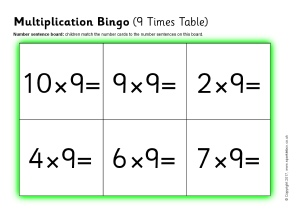Multiplication Bingo (9 Times Table) (SB12048)

A set of printable bingo boards and number cards with multiplication number sentences from the 9 times table.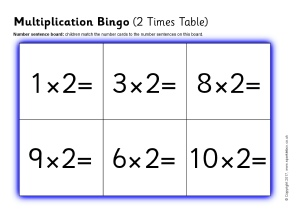Multiplication Bingo (2 Times Table) (SB12056)

A set of printable bingo boards and number cards with multiplication number sentences from the 2 times table.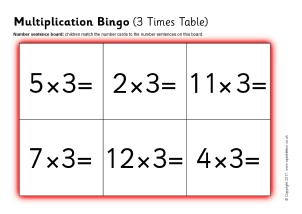Multiplication Bingo (3 Times Table) (SB12057)

A set of printable bingo boards and number cards with multiplication number sentences from the 3 times table.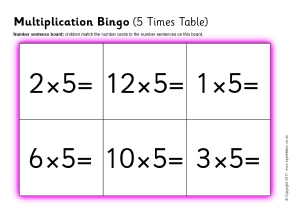Multiplication Bingo (5 Times Table) (SB12058)

A set of printable bingo boards and number cards with multiplication number sentences from the 5 times table.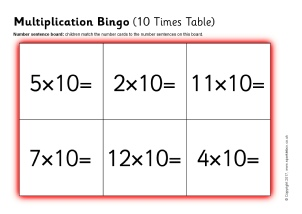Multiplication Bingo (10 Times Table) (SB12059)

A set of printable bingo boards and number cards with multiplication number sentences from the 10 times table.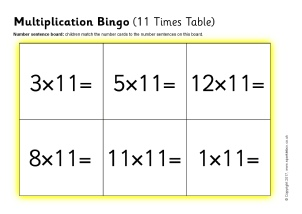Multiplication Bingo (11 Times Table) (SB12060)

A set of printable bingo boards and number cards with multiplication number sentences from the 11 times table.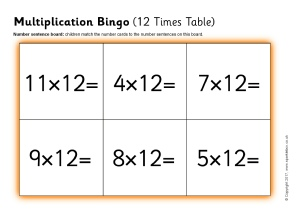Multiplication Bingo (12 Times Table) (SB12061)

A set of printable bingo boards and number cards with multiplication number sentences from the 12 times table.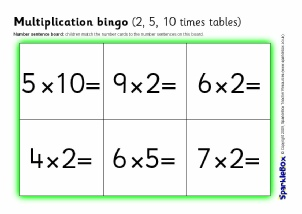Multiplication Bingo (2, 5, 10 Times Tables) (SB2341)

A set of printable bingo boards and number cards with multiplication number sentences.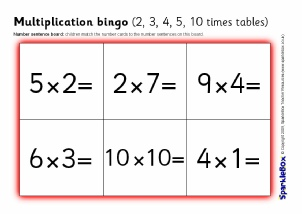Multiplication Bingo (2, 3, 4, 5 & 10 Times Tables) (SB6632)

A set of printable bingo boards and number cards with multiplication number sentences.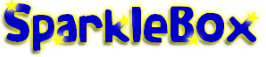New Literacy Maths Signs and Labels Class Management Special Needs Other Topics